# Ch6 编译原理 线性数据结构和位运算

Posted by R1NG on November 4, 2020 Viewed Times

# Ch6 线性数据结构和位运算

Motivation:

1. 使用合适的寻址模式访问线性数据结构
2. C 语言的字符串
3. 位运算和旋转
4. 数组

## 1. 字符串和寻址模式

### 1.1 字符串

Python 型字符串中包含字符和一些额外信息, 如字符串长度, 字符串哈希值等.

C 型字符串包含字符, 每个字符长为一个字节 ($8$ 位), 并且最后以 ** 数字 $0$** 结尾. (注意: 不是字符 $’0’$!)

1. String.length

计算某个字符串的长度:

For Python:

1
len = len(message)


For C:

1
2
3
len = 0;
for (i = 0; len[i] != 0; i++);
len = i;


For ARM Assembly (NOT Optimised):

1
2
3
4
5
6
7
8
MOV     R2, #0          ; R2 used as the loop counter i
again   LDRB    R0, [R1,R2]     ; Register offset addressing
CMP     R0, #0
BNE     again
SUB     R2, R2, #1      ; Why? Can this be avoided?
STR     R2, length


For ARM Assembly (Optimized):

1
2
3
4
5
6
7
MOV     R2, #0          ; R2 = i
again   LDRB    R0, [R1, R2]    ; Register offset
CMP     R0, #0
BNE     again
STR     R2, length


For ARM Assembly (Optimized for register use)

1
2
3
4
5
6
7
8
9
10
; Use Post-Indexed and save a register
ADDRL   R1, message       ; Pointer to a char
again   LDRB    R0, [R1], #1      ; Post Increment
CMP     R0, #0            ; Look for 0
BNE again
SUB     R0, R1, R0
SUB     R0, R0, #1
STR     R0, length
; Saved a register, but less concise or readable...


1. String.indexOf
在给定字符串中寻找给定字符第一次出现的位置:

For Python:

1
msg.find(‘e’)


For ARM Assembly:

1
2
3
4
5
6
7
8
again   LDRB    R0, [R1], #1
CMP     R0, #’e’          ;look for ’e’ instead of 0
BNE     again
SUB     R0, R1, R0
SUB     R0, R0, #1
STR     R0, find


### 1.2 列表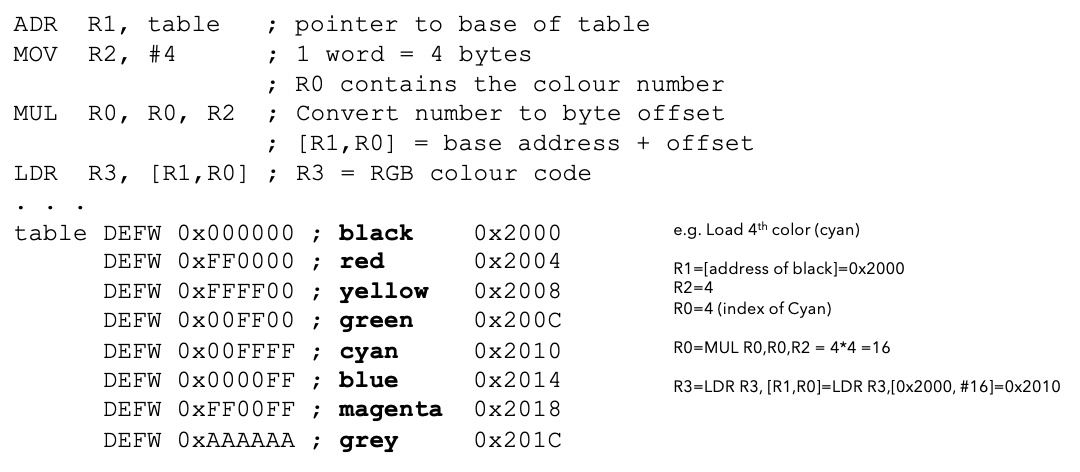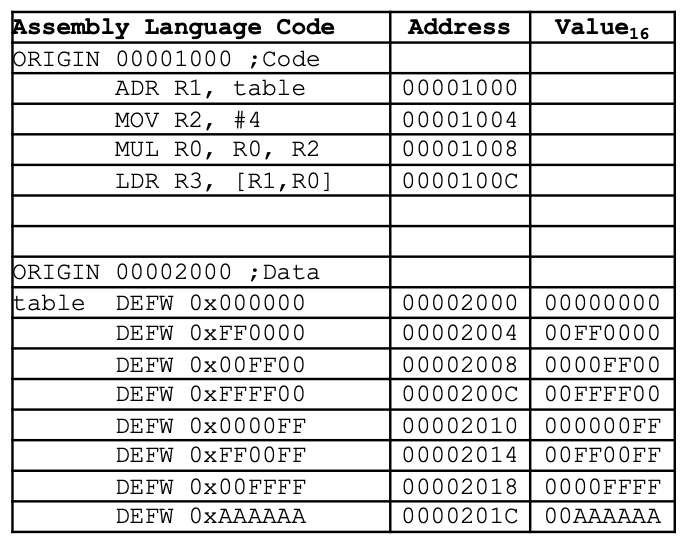## 2. 位运算

### 2.1 位运算 - 逻辑运算

1. and 按位与运算
运算规则和逻辑与运算相同, 用于二进制取位操作:
[例] 对一个二进制整数 and 1 的结果就是取该二进制整数的最末位. 所有的二进制奇数最低位均为 $1$, 而二进制偶数的最低位均为 $0$. 通过取某个二进制整数的最低位, 我们可以立即判断该数字的奇偶性.

[例]

1
2
3
; Rn = 0b11001100, Op2=0b00001111
AND Rd, Rn, Op2      ; Rd = 0b00001100
; clear all bits except those set in Op2

2. or 按位或运算
运算规则和逻辑或运算相同.
[例] or 运算通常用于对二进制整数的特定位进行无条件赋值. 如, 对一个二进制整数 or 1 的结果即为将其末位强行变为 $1$. 利用这一原理, 我们可以使用 or 运算, 通过对其和 $1$ 执行一次 or 运算后再减去 $1$, 即可将一个二进制整数变为最接近它的偶数.

[例]

1
2
3
4
5
; Rn = 0b11001100, Op2=0b00001111
ORR Rd, Rn, Op2      ; Rd = 0b11001111
BIC Rd, Rn, Op2      ; Rd = 0b11000000
; Set those bits that are set in Op2: any bit in Op2 that is 1, is set to  in Rd
; Or CLEAR those bits that are set in Op2

3. not 按位非运算
运算规则和逻辑非运算相同. 由于其定义即为将被操作数的每个位全部取反, 因此在进行按位非运算时需要注意被操作数是否为含符号整数. 若被执行数为一个无符号二进制整数, 则得到的结果即为该数和该类型上界的差.

4. xor 按位异或运算
运算规则和逻辑异或运算相同.
[例] 按位异或运算常用于对二进制整数的特定位进行取反操作. 并且, 取反运算与其本身互为逆运算, 亦即以任何一个二进制整数和另一个整数作为操作符, 连续执行两次异或运算的结果为其本身. 因此, 异或运算可用于简单的加密:
1
2
3
114514 ^ 1919810 = 1897488
1897488 ^ 114514 = 1919810
1897488 ^ 1919810 = 114514


此外, 我们还可以使用异或运算进行变量的交换:

1
2
3
4
def swap(x, y)
x = x ^ y
y = x ^ y
x = x ^ y

5. LSL 逻辑左移位运算
该运算由两个操作符组成: a LSL b 即为: 将二进制整数 $a$ 左移 $b$ 位. 我们常用逻辑左移代替涉及 $2^n$ 的乘法运算.
[例] 11011111101010010 (bin) = 114514 (dec), 114514 LSL 2 = 458056, 亦即: a LSL b = a * 2^b.

6. LSR 逻辑右移位运算
和逻辑左移位运算相似, 逻辑右移位运算表示将二进制整数向右移位, 相当于将二进制整数除以 $2$ 的幂, 常用于代替特定的除法运算.

### 2.4 ARM 移位指令

LSL #n 逻辑左移 $n$ 位 将有符号或无符号二进制整数乘以 $2^n$
LSR #n 逻辑右移 $n$ 位 将无符号二进制整数除以 $2^n$
ROR #n 右旋转 $n$ 位 旋转位: lsb $\rightarrow$ Carry
RRX #n 右旋转并扩展 $n$ 位 围绕 Carry 位旋转位

### 2.5 逻辑运算

ARM 汇编语言中的布尔逻辑运算的定义, 运算类型和运算优先级与 Python 一致. 我们需要使用八个布尔值才能表示一个字, 但在涉及一些特殊的运算操作, 如从这个字中取出某一个特定位时, 如果这个字是用布尔值表示的, 那么我们只需要将其和一个特定的 $8$ 位无符号整数做与运算即可得知这个位上是 $1$ 还是 $0$. (此处不做解释, 自己思考一下应该如何实现)

### 2.6 ARM 位运算

ARM 架构中的逻辑运算或布尔运算是以位运算的形式实现的:

1
2
3
4
5
; assume R1 = 0b11001100 R2 = 0b00001111
AND R0, R1, R2  ;R0 = 0b00001100      (AND)
ORR R0, R1, R2  ;R0 = 0b11001111      (OR)
EOR R0, R1, R2  ;R0 = 0b11000011      (XOR)
MVN R0, R2      ;R0 = 0b11110000      (NOT)  invert R2, store to R0


1
2
3
4
5
6
7
8
9
10
11
12
13
14
15
16
17
; 1.
; notice that although the 8-digit num has 0s on other higher bits, it is still considered as an 8-bit num instead of a 1-bit num!!
!00000001 == 11111110 != 0

; 2.
; assume we want to describe a formula:
; b4 = b1 & b2 | !b3
; take care that we must follow the operator order:

LDRB R0, b1
LDRB R1, b2
AND  R0, R0, R1  ;do b1&b2 first
LDRB R1, b3
EOR  R1, R1, #1  ;b3^1 = !b3
;for better performance, we wisely use decimal arithmetic quirks here
ORR  R0, R0, R1  ;b1&b2 | !b3
STRB R0, b4      ;store the final result


ARM 架构提供了对每一个位执行 无条件赋值, 清除 (亦即将其值无条件赋值为 $0$), 和测试其值的方法.

1
2
3
4
; Rn = 0b11001100, Op2=0b00001111
TST Rn, Op2
; TST == 'Test': run Rn AND Op2, sets th flags, but discard the result.
; Op2 can be a reg, immediate value, shifted value, rotated value, etc.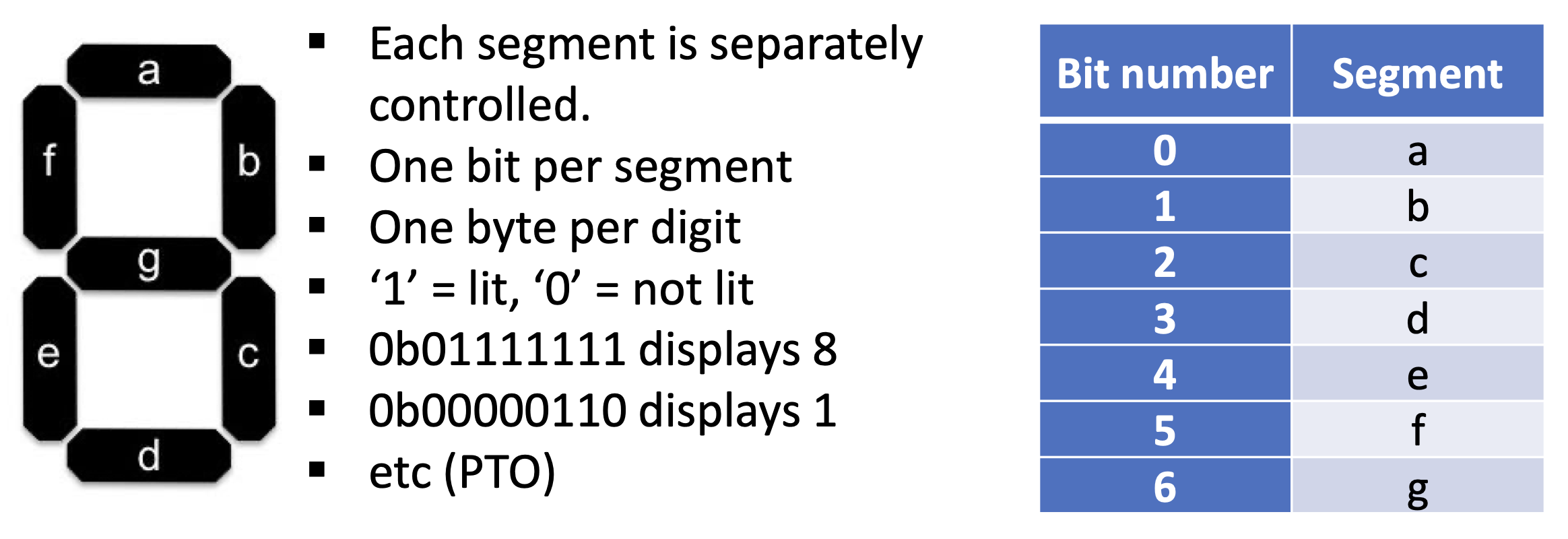1
2
3
4
5
6
7
8
LDRB    R2, [R1, R0]
STRB    R2, display

segments: 00111111      ; display 0
00000110      ; disply 1
01011011      ; display 2
......


1
AND     R2, R0, #0b1111


### 2.6 短路特性

1
2
3
4
5
6
7
8
9
10
11
b = (x<y and y<z) or (x>z)

we can rewrite to test x<y first:

if (x<y): b = (True and y<z) or (x>z)           # if x<y True
else: b = (False and y<z) or (x>z)              # if x<y False

equivalent to:

if (x<y):       b = (y<z) or (x>z)
else:           b = x>z


1. 与运算具有最直接的短路特性: 只要其中一个条件为假, 则整个表达式必为假:
2. 或运算同样具有短路特性: 只要其中一个条件为真, 则整个表达式就为真.

## 3. 数组

### 3.1 数组的定义, 访问和存储

[例]

ARM 汇编中, 定义数组的方法如下:

1
2
3
4
5
6
7
8
9
10
11
12
13
14
; Array of student heights
; Note: single byte per student: +ve integer heights <256cm
; Note: the program does not have a zeroth student
HEIGHTS    DEFB 0,150,160,165,155,150,170,175,180,155,160

; Set up base address and index
MOV        R2, #10       ;Index set to student number 10
ADR        R4, HEIGHTS   ;Make R4 the pointer to the array

; Access element 10 o the array using indexed addressing
MOV        R2, #10       ;Set the current student num to 10
LOOP    LDRB       R1, [R4, R2]  ;Height is at base address + I



1
2
for i in range(1, 10):
print(a[i])


1
LDRB    R1, [R4], #1       ;fetch [R4], then R4 += 1


1
2
3
4
5
6
LDRB    R1, [R4, R2]            ;for array of bytes

; and...

LDR     R1, [R4, R2, LSL #2]    ;for array of 4-byte words
; do logical shift left by 2, multiply the difference by 4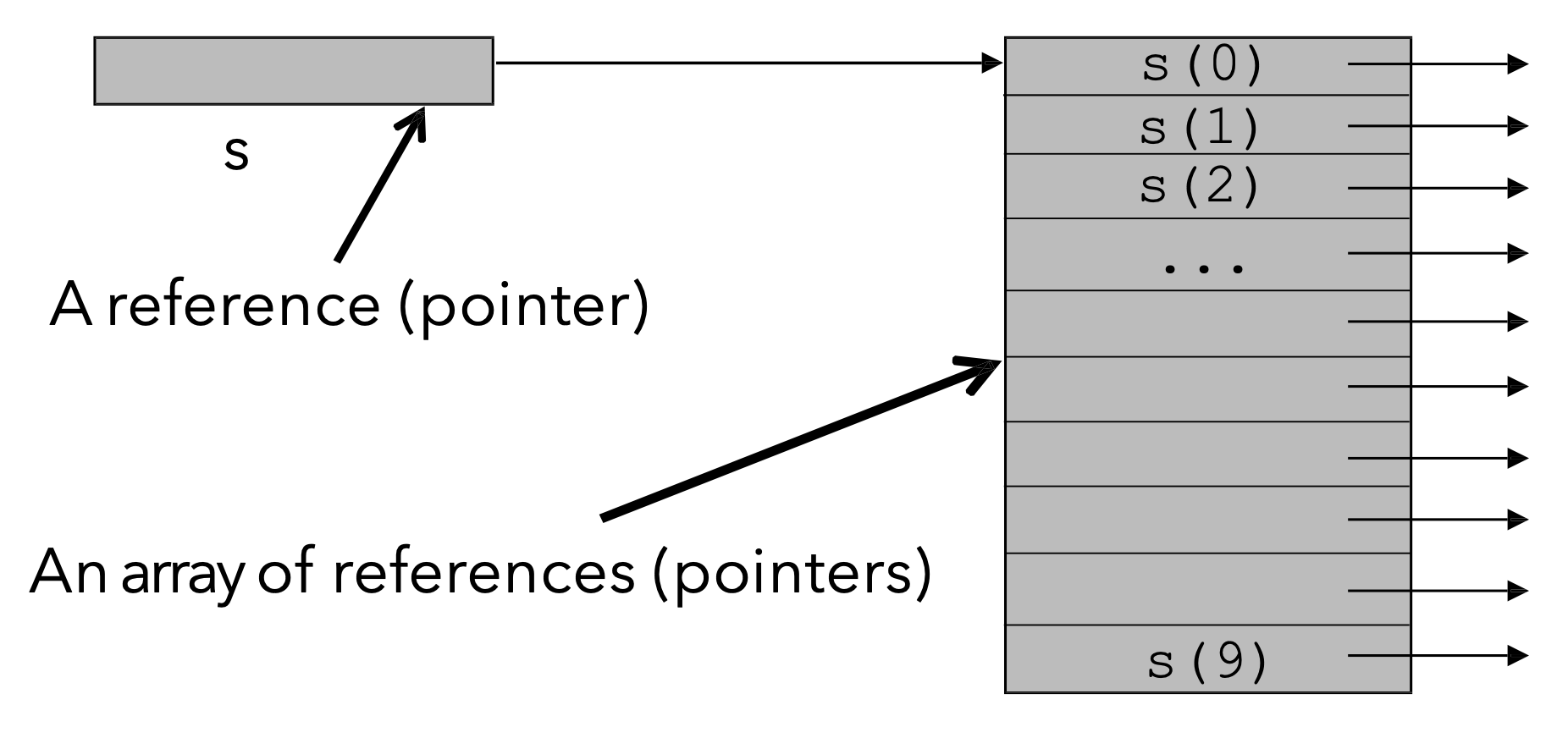1
s = "abcd"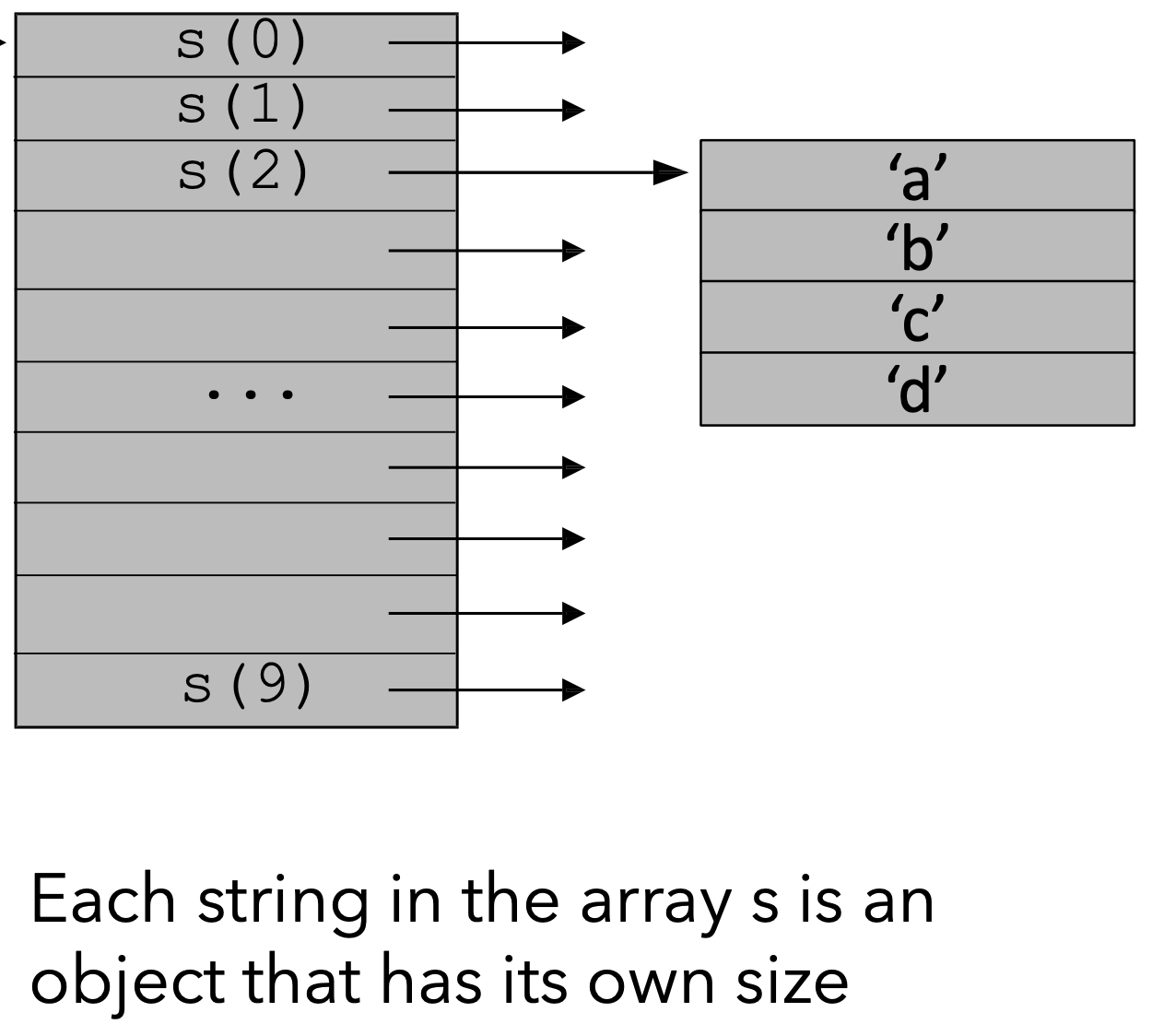### 3.2 高维数组

1
m = [[0, 1, 2, 3], [4, 5, 6, 7]]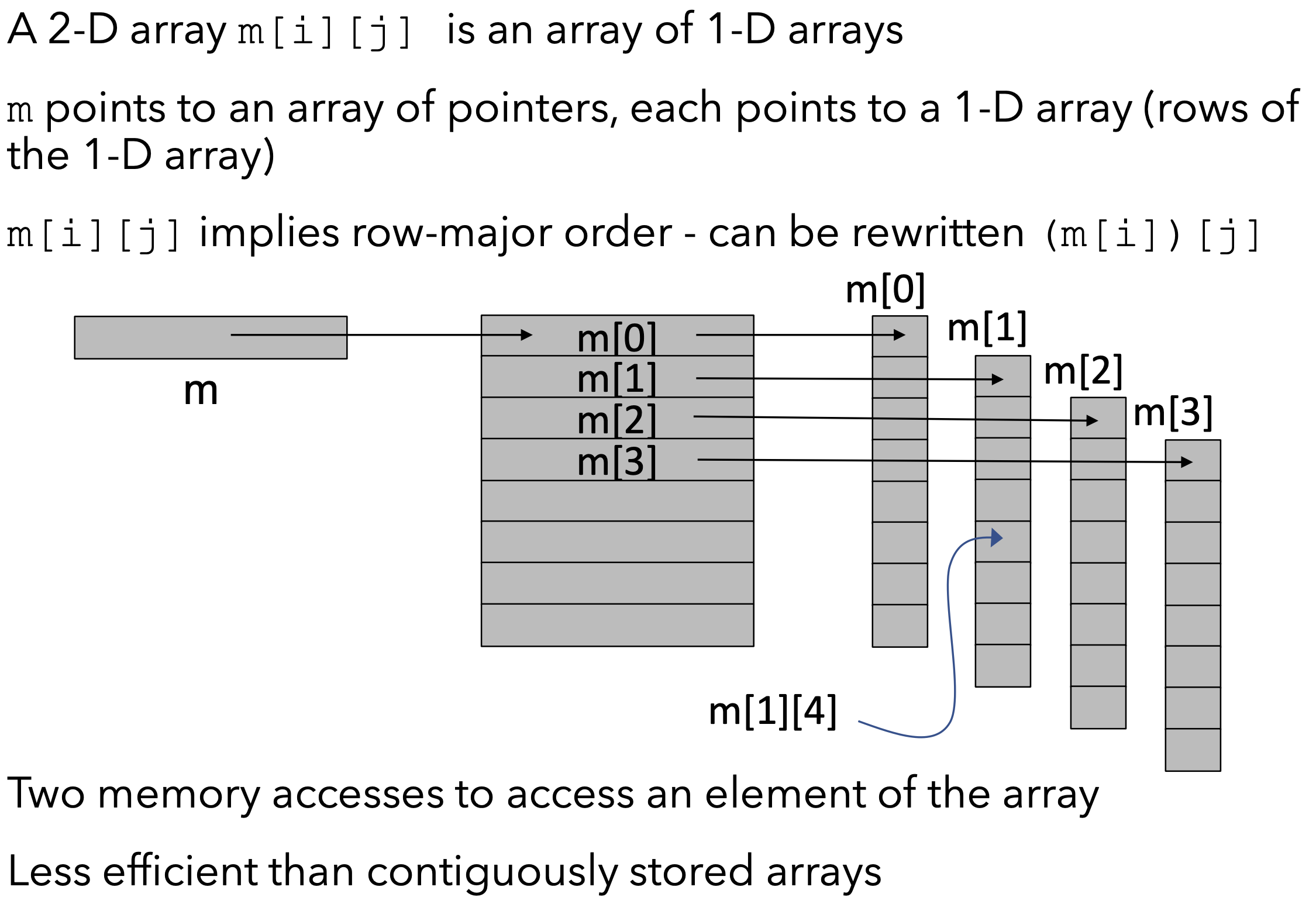### 3.3 数组/内存边界检测

1
2
3
4
5
6
7
; assume we have the code b = a[x * x]
; R2 is the pointer which contains the base address
; R1 is used as the index, R0 contains x
MUL R1, R0, R0                  ; x * x
CMP R1, #N;                     ; compare R1 and bound N
BGE error                       ; handle exception if index > N
LDR R3, [R2, R1, LSL #2]        ; 32 bit
`